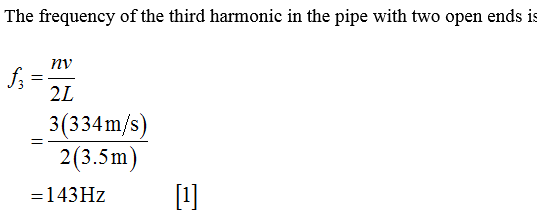# Consider standing waves in a pipe of length L = 3.5 m containing a column of air. The speed of sound in the columns is vs = 334 m/s. Each of the standing wave images provided may represent a case for which one or both ends are open.Select the image from the options provided showing the displacement of air particles for the third harmonic of a pipe with two open ends.  Calculate the wavelength λ3 in m for the third harmonic in the pipe with two open ends. Calculate the frequency f1 in Hz for the fundamental harmonic in the pipe with two open ends.

Question
30 views

Consider standing waves in a pipe of length L = 3.5 m containing a column of air. The speed of sound in the columns is vs = 334 m/s. Each of the standing wave images provided may represent a case for which one or both ends are open.

Select the image from the options provided showing the displacement of air particles for the third harmonic of a pipe with two open ends.

Calculate the wavelength λ3 in m for the third harmonic in the pipe with two open ends.

Calculate the frequency f1 in Hz for the fundamental harmonic in the pipe with two open ends.

check_circle

Step 1...

### Want to see the full answer?

See Solution

#### Want to see this answer and more?

Solutions are written by subject experts who are available 24/7. Questions are typically answered within 1 hour.*

See Solution
*Response times may vary by subject and question.
Tagged in